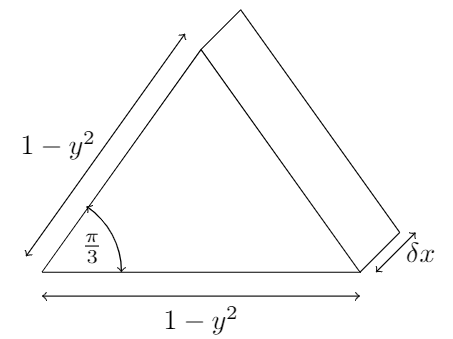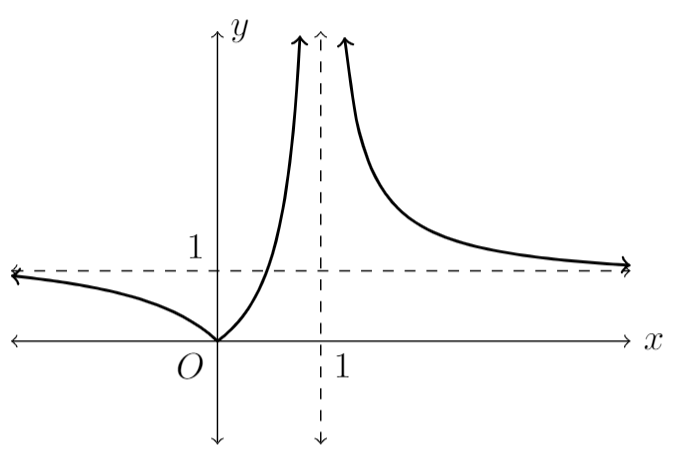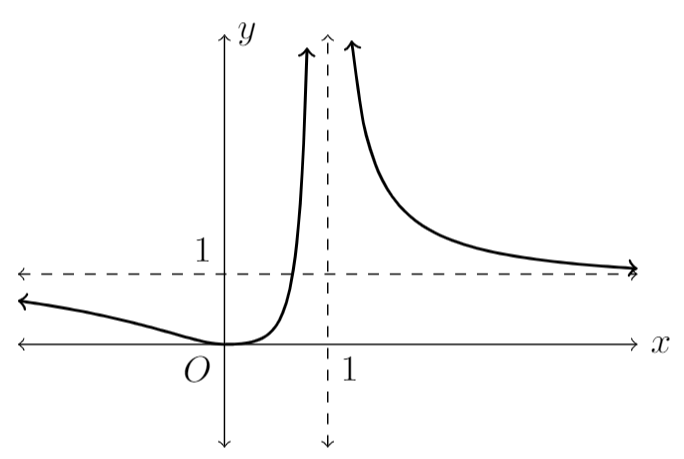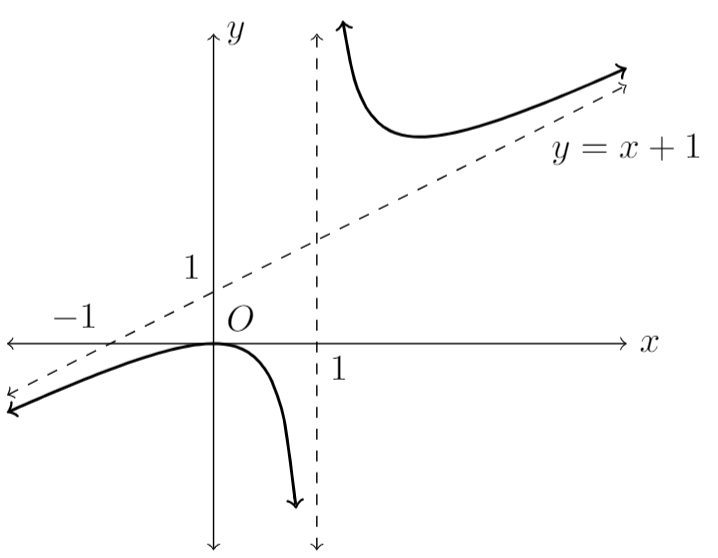# 2018 HSC Maths Ext 2 Exam Paper Solutions

In this post, we give you the solutions to the 2018 Maths Extension 2 paper.Have you seen the 2018 HSC Mathematics Extension 2 Paper yet? In this post, we will work our way through the 2018 HSC Maths Extension 2 paper and give you the solutions, written by our leading Mathematics teacher Oak Ukrit and his team.

Read on to see how to answer all of the 2018 questions.

## 2018 HSC Mathematics Extension 2 Exam Paper Solutions (25.10.2018)

### Multiple Choice

1. (B) Standard $$\sin^{-1}$$ integral

2. (C) Rearrange the given equation into the form $$\frac{x^2}{a^2} – \frac{y^2}{b^2} = 1$$, Asymptotes are $$y = \pm\frac{b}{a}x$$.

3. (D) Let $$x = -\frac{1}{\alpha} \Rightarrow \alpha = -\frac{1}{x}$$. Substitute into the original equation then rearrange.

$$P(x) = x^3 + 5x^2 – 2x + 1 = 0$$

4. (C) Since the graph must be positive, this results in either (A) or (C), as $$x$$ approaches infinity, $$y$$ approaches zero, so the answer is (C).

5. (A) Use disc method, centered at $$y = -1$$. The radius of each disc is $$e^{3x} + 1$$, apply integral to find the volume.

6. (A) Standard root of unity question. $$z^6 = r^6\text{cis}(6\theta)$$. Find general solution where $$6\theta = \frac{\pi}{2} + 2k\pi$$ where $$k = 0,\pm 1,\pm 2,3$$, or convert each option to polar form and raise to power of $$6$$, to find that (A) is the solution.

7. (D) Rearrange the equation to obtain

$$\arg(z) – \arg(z – (-1 + i)) = 0$$

This is a special case where both vectors must point in the same direction as the difference of the angles between both vectors must be zero, resulting in (D).

8. (B) Given a gradient function. Conditions for point of inflexion of original function is $$\frac{d^2y}{dx^2}=0$$ and that $$\frac{d^2y}{dx^2}$$ has to change signs between two points adjacent to it. Only (B) satisfies this condition (D) does not.

9. (B) Let $$p = ic$$ and $$q = id$$

$$-a^2c^2 – b^2d^2 \leq -2abcd$$
$$a^2b^2 + c^2d^2 \geq 2abcd$$

10. (C) We know that the turning points of $$f(x) = \sin x$$ occur at $$x = \frac{\pi}{2} + k\pi$$, where $$k \in \mathbb{Z}$$. For the stationary points of $$g(x) = x\sin x$$, we obtain the equation

$$g'(x) = 0$$
$$\sin x + x\cos x = 0$$
$$therefore \tan x = -x$$

Thus the turning points of $$g(x)$$ must occur at the solutions to that equation. To see the positions of the solutions, we must solve graphically. After graphing $$\tan x$$ and $$-x$$ simultaneously, notice that the points of intersection occur close to the asymptotes of $$\tan x$$, which are where the turning points of $$f(x) = \sin x$$ occur. As these close intersection points are to the right of the asymptote when $$x$$ is positive and to the left when $$x$$ is negative, we must have that $$|a| < |b|$$.

### Written Responses

11. (a) i.

$$zw = (2+3i)(1-i)$$
$$= 2-2i+3i+3$$
$$= 5+i$$

11. (a) ii.

$$\bar{z} – \frac{2}{w}= (2-3i) – \frac{2\bar{w}}{w\bar{w}}$$
$$= (2-3i) – \frac{2(1+i)}{1+1}$$
$$= 1 – 4i$$

11. (b)

$$p(r)=r^3 + ar^2 + b = 0$$
$$p(4) = 4^3 + 4^2\times a + b = 0$$
$$p'(x) = 3x^2 + 2ax$$
$$p'(4) = 3\times16 + 8a = 0$$
$$\Rightarrow a = -6$$
$$\Rightarrow b = -4^3-16a = 32$$
$$\prod \text{roots} = -b = 4^2 \times r$$
$$r = -\frac{b}{16} = -2$$

11. (c)

\begin{align*}
x^2-x-6\equiv a(x^2-3) + (bx+c)(x+1) \\
\text{setting $x$ = -1 gives } -4 = -2a \\
a = 2 \\
\text{equating coefficients of $x^2$: } 1=a+b \\
b=1-a \\
= -1 \\
\text{equating coefficients of $x$: } b+c=-1 \\
c=-1-b \\
= 0 \\
\text{so } \frac{x^2-x-6}{(x+1)(x^2-3)}\equiv \frac{2}{x+1} – \frac{x}{x^2-3} \\
\int{\frac{x^2-x-6}{(x+1)(x^2-3)}dx} = \int{\frac{2}{x+1} – \frac{x}{x^2-3} dx} \\
= 2\ln{|x+1|}-\frac{1}{2}\ln{|x^{2}-3|}+C
\end{align*}

11. (d) i.

$$w = i\times u = i(5+2i) = -2+5i \\$$

11. (d) ii.

$$v = u+w = (5+2i) + (-2+5i) = 3 + 7i \\$$

11. (d) iii.

$$\arg\left(\frac{w}{v}\right) = \arg{w} – \arg{v} \\ = \frac{\pi}{4} \text{ (diagonal of square)}$$

11. (e)

$$\text{Construct CB} \\ \Rightarrow \angle ACB = 90° \; \text{(angle in a semicircle)}\\ \text{also, } \angle D = \angle ADC \\ = \angle ABC \text{(angles subtended by common arc AC are equal)} \\ \text{Now, } \frac{d}{AB} = \sin(\angle ABC) \\ = \sin(\angle D) \\ d = 2r\sin(D)$$

12. (a)$$\delta V = \frac{1}{2}(1-y^2)^2 \sin(\frac{\pi}{3}) \delta y \\ = \frac{\sqrt{3}}{4}(1-y^2)^2 \delta y \\ V = 2 \int_{0}^{1}{\frac{\sqrt{3}}{4}(1-y^2)^2 dy} \\ = \frac{\sqrt{3}}{2} \int_{0}^{1}{1-2y^2+y^4 dy} \\ = [\frac {\sqrt{3}{2}} y – \frac{2}{3}y^3 + \frac{1}{5}y^5 ]_{0}^{1} \\ = \frac{\sqrt{3}}{2} \times \frac{8}{15} \\ = \frac{4\sqrt{3}}{15} \text{units}^3 \\$$

12. (b) i.

$$x^2 + xy + y^2 = 3 \\ 2x + y + x\frac{dy}{dx} + 2y\frac{dy}{dx} = 0 \\ \frac{dy}{dx}(x+2y) = -(2x + y) \\ \frac{dy}{dx} = -\frac{2x+y}{x+2y}$$

12 (b) ii.

$$\frac{dy}{dx} = 0 \Rightarrow -\frac{2x+y}{x+2y} = 0 \\ 2x + y = 0 \\ y = -2x \\ \text{Substituting into original equation: } x^2 + x(-2x)+(-2x)^2 = 3 \\ 3x^2 = 3 \\ x = \pm 1 \\ \text{So, } y = \mp 2 \\ \Rightarrow \frac{dy}{dx} = 0 \; \text{at} \; (1, -2) \; \text{and} \; (-1,2) \\$$

12. (c)

$$\int{\frac{x^2+2x}{x^2+2x+5}dx} = \int{\frac{x^2+2x + 5 – 5}{x^2+2x+5}dx} \\ = \int{1-\frac{5}{x^2+2x+5}dx} \\ = \int{1-\frac{5}{(x+1)^2+4}dx} \\ = x – \frac{5}{2}\tan^{-1}\left(\frac{x+1}{2}\right) + C$$

12. (d) i.12. (d) ii.12. (d) iii.13. (a)

$$V = 4\pi \int_{0}^{1}{\sqrt{x}(1-x)^2dx} \\ = \frac{64}{105}\pi \; \text{units}^3 \\$$

13. (b) i.

$$|z| = \sqrt{(1-\cos(2\theta))^2+\sin^2(2\theta)} \\ = \sqrt{1+\cos^2 2\theta + \sin^2 2\theta – 2\cos 2\theta} \\ = \sqrt{2(1-\cos 2\theta)} \\ = \sqrt{2(2\sin^2 \theta)} \\ = 2\sin\theta \\ \text{note \sin\theta > 0 as 0 < \theta < \pi}$$

13. (b) ii.

$$\arg(z) = \tan^{-1}\left( \frac{\sin 2\theta}{1-\cos 2\theta} \right) \\ = \tan^{-1}\left( \frac{2\sin\theta\cos\theta}{2\sin^2 \theta} \right) \\ = \tan^{-1}\left( \frac{\cos\theta}{\sin\theta} \right) \\ = \tan^{-1}\left( \cot\theta \right) \\ = \tan^{-1}\left( \tan(\frac{\pi}{2}-\theta) \right) \\ \text{As 0<\theta\leq \pi, so \frac{\pi}{2}-\theta \in [-\frac{\pi}{2},\frac{\pi}{2})} \\ \text{So, } \tan^{-1}\left( \tan(\frac{\pi}{2}-\theta) \right) = \frac{\pi}{2} – \theta \\$$

13. (c)

$$\text{Horizontal component of tension: } \\ T_x = m\omega^2 r \\ \Rightarrow T = \frac{T_x}{sin\theta} = \frac{m\omega^2 r}{sin\theta} \\ \text{Vertical component of tension: } \\ T_y = T\cos\theta = m\omega^2 r \cot \theta \\ \text{For the particle to not leave table, } T_y \leq mg \\ m\omega^2 r \cot\theta \leq mg \\ \omega^2 \leq \frac{g\sin\theta}{r \cos\theta} \\ \text{since l = \frac{r}{\sin\theta}} \\ \omega^2 \leq \frac{g}{l\cos\theta}$$

13. (d) i.

$$\text{x-intercept of PQ (y=0): } R = (c(p+q),0) \\ \text{y-intercept of PQ (x=0): } S = \left(0,\frac{c(p+q)}{pq}\right) \\ = \left(0,\frac{c}{p} +\frac{c}{q} \right) \\ \Rightarrow |SP|^2 = (cp)^2 + \left(\frac{c}{p} – \left(\frac{c}{p} +\frac{c}{q} \right)\right) \\ =(cp)^2 + \left( \frac{c}{q} \right)^2 \\ \Rightarrow |QR|^2 = (c(p+q)-cq)^2 + \left( \frac{c}{q} \right)^2 \\ =(cp)^2 + \left( \frac{c}{q} \right)^2 \\ \Rightarrow |SP| = |QR| \\$$

13. (d) ii.

$$\text{Let the tangent have x and y intercepts R and S} \\ \text{By (i), } |BS| = |AR| \\ \text{M is the midpoint of AB} \Rightarrow |AM| = |MB| \\ |MR| = |AM|-|AR| = |BM|-|BS|=|MS| \\ \text{So M is also the midpoint of RS} \\ \text{Now the tangent y=tx-at^2 has a y intercept (0,-at^2) and an x intercept (at, 0)} \\ \Rightarrow M = \left(\frac{at}{2},-\frac{at^2}{2} \right) = (x_m,y_m) \; \text{ say} \\ \text{we want coordinates to satisfy 2x^2 = -ay} \\ \text{LHS} = 2x_m^2 = 2\left(\frac{at}{2}\right)^2 = \frac{a^2t^2}{2}\\ \text{RHS} = -ay_m = -a\left(-\frac{at^2}{2}\right) = \frac{a^2t^2}{2} = \text{LHS}\\ \text{So M\left(\frac{at}{2},-\frac{at^2}{2}\right) does lie on the required parabola}$$

14. (a)

$$Let \ t = \tan\frac{\theta}{2}$$

$$dt = \frac{1}{2}\sec^2\frac{\theta}{2}d\theta \\ \frac{2}{1 + t^2}dt = d\theta \\ \theta = \frac{\pi}{2} \Rightarrow t = 1 \\ \theta = 0 \Rightarrow t = 0 \\ \int_{0}^{\frac{\pi}{2}}\frac{1}{2 – \cos\theta}\,d\theta = \int_0^1\frac{1}{2 – \frac{1 – t^2}{1+t^2}} \times \frac{2}{1+t^2}\, dt \\ = \int_0^1\frac{2}{2(1+t^2) – (1-t^2)}\,dt \\ = \int_{0}^1\frac{2}{1+3t^2}\,dt \\ = \left[\frac{2}{\sqrt{3}}\tan^{-1}(\sqrt{3}t)\right]^1_0 = \frac{2}{\sqrt{3}}\times\frac{\pi}{3} = \frac{2\pi}{3\sqrt{3}}$$

14. (b)

$$\text{at } t = 0, v = 0, x = 0$$

\begin{align*}
a = g – kv^2 \\
v\frac{dv}{dx} = g – kv^2 \\
\int \frac{v}{g – kv^2}dv = \int 1\,dx \\
-\frac{1}{2k}\ln(g-kv^2) = x + C
\end{align*}
\begin{gather*}
\text{at } x = 0, v = 0, C = -\frac{1}{2k}\ln(g) \\
\text{therefore} -\frac{1}{2k}\ln\left(\frac{g-kv^2}{g}\right) = x
\end{gather*}

$$\text{at} \ x=h, \frac{g-kv^2}{g} = e^{-2kh} \\ kv^2 = g -ge^{-2kh} \\ v^2 = \frac{g}{k}(1-e^{-2kh}) \\ v = \sqrt{\frac{g}{k}(1-e^{-2kh})} \quad \text{since } v > 0$$

14. (c). i.

\begin{gather*}
I_n = \int_{-3}^0x^n\sqrt{x+3}\,dx \\
U = x^n \quad dV = \sqrt{x+3}\,dx \\
dU = nx^{n-1}dx \quad V = \frac{2}{3}(x+3)^{\frac{3}{2}}
\end{gather*}
\begin{align*}
I_n &= \left[\frac{2}{3}x^n(x+3)^{\frac{3}{2}}\right]^0_{-3} – \frac{2}{3}\int_{-3}^0nx^{n-1}(x+3)\sqrt{x+3}\,dx \\
I_n &= 0 – \frac{2n}{3}\int_{-3}^0\left(x^n\sqrt{x+3} + 3x^{n-1}\sqrt{x+3}\right)\,dx \\
I_n &= -\frac{2n}{3}\int_{-3}^0x^n\sqrt{x+3} + 3x^{n-1}\sqrt{x+3}\,dx \\
&= -\frac{2n}{3}I_n – 2nI_{n-1} \\
I_n\left(\frac{3 + 2n}{3}\right) &= -2nI_{n-1} \\
\text{therefore} \ I_n &= -\frac{6n}{3+2n}I_{n-1}
\end{align*}

14. (c) ii.

\begin{align*}
I_2 &= -\frac{12}{7}I_1 \\
&= -\frac{12}{7} \times -\frac{6}{5}I_0 \\
&= \frac{72}{35}\left[\frac{2}{3}(x+3)^{\frac{3}{2}}\right]_{-3}^0 \\
&= \frac{144\sqrt{3}}{35}
\end{align*}

14. (d) i.

$$\Pr(\text{A only}) = \left(\frac{1}{3}\right)^n$$

14. (d) ii.

\begin{align*}
\Pr(\text{no C}) = \left(\frac{2}{3}\right)^n \\
\Pr(\text{A only}) = \Pr(\text{B only}) = \left(\frac{1}{3}\right)^{n} \\
\text{therefore} \Pr(\text{A or B or C}) = \Pr(\text{no C}) – \Pr(\text{A only}) – \Pr(\text{B only}) \\
= \left(\frac{2}{3}\right)^n – 2\left(\frac{1}{3}\right)^n \\
\end{align*}

14. (d) iii.

\begin{align*}
\Pr(\text{at least one each}) = 1 – \Pr(\text{A only}) – \Pr(\text{B only}) – \Pr(\text{C only}) – \Pr(\text{A or B not C}) \\
\,\,\,\,\,\,- \Pr(\text{B or C not A}) – \Pr(\text{A or C not B}) \\
= 1 – 3\left(\frac{1}{3}\right)^n – 3\left(\left(\frac{2}{3}\right)^n – 2\left(\frac{1}{3}\right)^n\right) \\
= 1 – 3\left(\frac{1}{3}\right)^n – 3\left(\frac{2}{3}\right)^n + 6\left(\frac{1}{3}\right)^n \\
= \frac{3^{n-1}-2^n +1}{3^{n-1}}
\end{align*}

15. (a) i.

\begin{align*}
\angle AOB = \frac{\pi}{2} \\
\text{therefore} \ B = \left(a\cos\left(\theta + \frac{\pi}{2}\right),a\sin\left(\theta + \frac{\pi}{2}\right)\right) \\
= (-a\sin\theta,a\cos\theta)
\end{align*}
So the $$x$$-coordinate of $$Q$$ is $$-a\sin\theta$$. Similarly the $$y$$-coordinate of $$Q$$ is $$b\cos\theta$$, which can be derived in the similar fashion as $$y_b$$.

15. (a) ii.

\begin{align*}
m_{OP} = \frac{b\sin\theta}{a\cos\theta} = \frac{b}{a}\tan\theta \\
m_{OQ’} = -\frac{b\cos\theta}{a\sin\theta} = -\frac{b}{a}\cot\theta \\
\text{therefore} \tan\left|\angle POQ’\right| = \left|\frac{\frac{b}{a}\tan\theta + \frac{b}{a}\cot\theta}{1 – \frac{b^2}{a^2}\tan\theta\cot\theta}\right| \\
= \left|\frac{ab\tan\theta + ab\cot\theta}{a^2 – b^2}\right| \\
= \left|\frac{ab}{a^2 – b^2}\right|\left(\frac{\sin\theta}{\cos\theta} + \frac{\cos\theta}{\sin\theta}\right) \\
= \frac{ab}{a^2 – b^2}\left(\frac{\sin^2\theta + \cos^2\theta}{|\sin\theta\cos\theta|}\right) \quad (a>b \text{ removes } |\cdot|) \\
= \frac{ab}{a^2 – b^2}\left(\frac{2}{|\sin2\theta|}\right)
\end{align*}
$$|\sin2\theta| \leq 1 \Rightarrow \frac{1}{|\sin2\theta|} \geq 1 \Rightarrow \tan|\angle POQ’| \geq \frac{2ab}{a^2 – b^2}$$
As equality occurs at $$\theta = \frac{\pi}{4}$$, $$\tan(\angle POQ’_\text{min}) = \frac{2ab}{a^2- b^2}$$. We note that $$y = \tan x$$ is monotonic and that $$\tan x$$ is minimised. Thus $$x$$ is minimised.
$$\text{therefore} \min(\angle POQ’) = \tan^{-1}\left(\frac{2ab}{a^2-b^2}\right)$$

15 (b) i.

$$(\cos\theta + i\sin\theta)^8 = \cos8\theta + i\sin8\theta$$
So for $$\sin8\theta$$, we need only the imaginary parts of the binomial. These occur at $$i,i^3,i^5,i^7$$. Hence we selectively choose these coefficients.
\begin{align*}
(\cos\theta + i\sin\theta)^8 = \sum_{k=0}^8 i^k \binom{8}{k} \sin^k\theta \cos^{8-k}\theta \\
\text{therefore} \ i\sin 8\theta = \binom{8}{1}i\cos^7\theta\sin\theta + \binom{8}{3}i^3\sin^3\theta\cos^5\theta + \binom{8}{5}i^5\sin^5\theta\cos^3\theta + \binom{8}{7}i^7\sin^7\theta\cos\theta \\
= i\left(\binom{8}{1}\cos^7\theta\sin\theta – \binom{8}{3}\sin^3\theta\cos^5\theta + \binom{8}{5}\sin^5\theta\cos^3\theta – \binom{8}{7}\sin^7\theta\cos\theta \right) \\
\text{therefore} \sin8\theta = \binom{8}{1}\cos^7\theta\sin\theta – \binom{8}{3}\sin^3\theta\cos^5\theta + \binom{8}{5}\sin^5\theta\cos^3\theta – \binom{8}{7}\sin^7\theta\cos\theta \quad \text{ as required.}
\end{align*}

15. (b) ii.

\begin{align*}
\frac{\sin8\theta}{\sin2\theta} = \frac{\binom{8}{1}\cos^7\theta\sin\theta – \binom{8}{3}\sin^3\theta\cos^5\theta + \binom{8}{5}\sin^5\theta\cos^3\theta – \binom{8}{7}\sin^7\theta\cos\theta}{2\sin\theta\cos\theta} \\
= \frac{\binom{8}{1}}{2}\cos^6\theta – \frac{\binom{8}{3}}{2}\cos^4\theta\sin^2\theta + \frac{\binom{8}{5}}{2}\cos^2\theta\sin^4\theta – \frac{\binom{8}{7}}{2}\sin^6\theta \\
= 4(1-\sin^2\theta)^3 – 28\sin^2\theta(1-\sin^2\theta)^2 + 28(1- \sin^2\theta)\sin^4\theta \\
= 4(1 – 10\sin^2\theta + 24\sin^4\theta – 16\sin^6\theta)
\end{align*}

15. (c) i.

\begin{align*}
LHS = x^n – 1 – n(x – 1) \\
= \left(\frac{x^n-1}{x-1}\right)(x-1) – n(x-1) \\
= (x-1)\left(\frac{x^n-1}{x-1} – n\right) \\
= (x-1)\left(1 + x + \cdots + x^{n-1} -n\right) \quad \text{by GP Formula}
\end{align*}

15. (c) ii.

Case 1, when $$x = 1$$,
$$LHS = 1^n = 1 \geq 0 = n(1-1) = 0 = RHS$$
Case 2, when $$0 < x < 1$$
\begin{gather*}
\text{therefore} \ x -1 < 0 \text{ and } x^k < 1 \text{ for } k \in \mathbb{Z}^+ \\
\text{therefore} \ 1 + x + \cdots + x^{n-1} – n < 0 \\
\text{therefore} \ (x -1)(1 + x + \cdots + x^{n-1} – n) \geq 0
\end{gather*}
Case 3, when $$x > 1$$
\begin{gather*}
\text{therefore} \ x -1 > 0 \text{ and } x^k > 1 \text{ for } k \in \mathbb{Z}^+ \\
\text{therefore} \ 1 + x + \cdots + x^{n-1} > 1 + \cdots + 1 = n \\
\text{therefore} \ 1 + x+ \cdots + x^{n-1} – n > 0\\
\text{therefore} \ (x -1)(1 + x + \cdots + x^{n-1} – n) \geq 0
\end{gather*}
Combining all 3 cases, we arrive at the desired inequality.

15 (c) iii.

\begin{gather*}
a^nb^{1-n} \geq na + (1-n)b \\
x^n \geq 1 + n(x – 1) \\
\end{gather*}
Substitute $$x = \frac{a}{b}$$:
\begin{align*}
\frac{a^n}{b^n} \geq 1 + n\left(\frac{a}{b} – 1\right) \\
= na + (1-n)b \\
\text{therefore} \ a^nb^{1-n} \geq na + (1-n)b \quad \text{as required.}
\end{align*}

16. (a)

Base Case $n = 1$:
\begin{gather*}
LHS = x^3 – 1 \\
RHS = (x-1)(x^2 + x + 1) = x^3 – 1 = LHS
\end{gather*}
Assume OK for $$n = k$$, i.e.
\begin{gather*}
x^{3^k} – 1 = (x-1)(x^2 + x + 1)\cdots(x^{2\times 3^{k-1}} + x^{3^{k-1}} + 1) \tag{*}
\end{gather*}
Let $$n = k+1$$: Want to show
$$x^{3^{k+1}} – 1 = (x-1)(x^2 + x + 1)\cdots(x^{2\times 3^{k}} + x^{3^k} + 1)$$
We go from $$RHS$$ to $$LHS$$:
\begin{align*}
RHS = \underbrace{(x – 1)(x^2 + x + 1)\cdots(x^{2\times 3^{k-1}} + x^{3^{k-1}} + 1)}_{\text{expression from } (*)}(x^{2\times 3^k} + x^{3^k} + 1) \\
= (x^{3^k} – 1)(x^{2\times 3^k} + x^{3^k} + 1) \\
= (x^{3^k})^3 – 1 \\
= x^{3^{k+1}} – 1 \\
= LHS \quad \text{as required.}
\end{align*}

16. (b) i

Note $$BDYH$$ is a parallelogram as opposite pairs of sides are parallel, so $$BH = DY$$. \\
Similarly, $$ZE = FC$$.

Now, $$\triangle CIH$$ is similar to $$\triangle CAB$$ with ratio $$\frac{1}{\sqrt{2}}$$.
\begin{gather*}
\sqrt{2} = \frac{BC}{HC} = \frac{BH + HC}{HC} = \frac{BH}{HC} + 1 \\
\text{therefore} \frac{BH}{HC} = \sqrt{2} – 1 \Rightarrow \text{therefore} \frac{DY}{HC} = \sqrt{2} – 1
\end{gather*}
Identical argument on $$\triangle BGF$$ and $$\triangle BAC$$
$$\text{therefore} \frac{CF}{HC} = \sqrt{2} – 1 \Rightarrow \frac{ZE}{HC} = \sqrt{2} – 1 = \frac{DY}{HC}$$
$$\text{therefore} \ ZE = DY$$

16. (b) ii.

\begin{align*}
\frac{YZ}{BC} = \frac{DE – DY – ZE}{BC} \\
= \frac{1}{\sqrt{2}} – 2\frac{DY}{BC} \quad (DY = ZE \text{ from above}) \\
\frac{HC}{DY} = \frac{1}{\sqrt{2}- 1}, \quad (\text{because} \ BH = DY) \\
\frac{BC}{DY} = \frac{HC + DY}{DY} = \frac{1}{\sqrt{2} – 1} + 1 = \frac{\sqrt{2}}{\sqrt{2} -1 } \\
\text{therefore} \frac{DY}{BC} = \frac{\sqrt{2} – 1}{\sqrt{2}} = 1 – \frac{1}{\sqrt{2}} \\
\text{therefore} \frac{YZ}{BC} = \frac{1}{\sqrt{2}} – 2\left(1 – \frac{1}{\sqrt{2}}\right) \\
= \frac{3}{\sqrt{2}} – 2 \\
\end{align*}

16. (c) i

By sum of roots, $$\alpha + \beta + \gamma = 0$$ \\
\begin{align*}
\text{therefore} \ \alpha = -(\beta + \gamma) \\
\alpha^2 = (\beta + \gamma)^2 = \beta^2 + 2\beta\gamma + \gamma^2 \\
\alpha^2 – 4\beta\gamma = \beta^2 – 2\beta\gamma + \gamma^2
\end{align*}
Now $$\beta\gamma = \frac{\alpha\beta\gamma}{\alpha} = \frac{-q}{\alpha}$$, note $$\alpha \not= 0$$ as $$q \not= 0$$.
$$\text{therefore} \ \alpha^2 + \frac{4q}{\alpha} = (\beta – \gamma)^2 \quad \text{as required.}$$

16. (c) ii.

\begin{align*}
(\beta- \gamma)^2 = \alpha^2 + \frac{4q}{\alpha} \\
= \frac{\alpha^3 + 4q}{\alpha} \\
= \frac{(\alpha^3 + p\alpha + q) + 3q – p\alpha}{\alpha} \\
= \frac{3q – p\alpha}{\alpha} \quad \text{since } \alpha^3 + p\alpha + q = p(\alpha) = 0
\end{align*}
Let $$x = (\beta – \gamma)^2$$
$$x = \frac{3q-p\alpha}{\alpha} \Rightarrow \alpha = \frac{3q}{x+p}$$
Substitute back into $$p(x)$$.
\begin{gather*}
\left(\frac{3q}{x+p}\right)^3 + p\left(\frac{3q}{x+p}\right) + q = 0 \\
q(x+p)^3 + 3pq(x+p)^2 + 27q^3 = 0
\end{gather*}
Now consider $$ax^3 + bx^2 + cx + d = 0$$
$$a = q, d = qp^3 + 3p^3q + 27q^3$$
\begin{align*}
(\alpha – \beta)^2(\beta – \gamma)^2(\gamma – \alpha)^2 = -\frac{d}{a} \\
= -\left(\frac{4p^3q + 27q^3}{q}\right) \\
= -(4p^3 + 27q^2)
\end{align*}

16. (c) iii.

\begin{align*}
27q^2 + 4p^3 < 0 \\
\Rightarrow -(27q^2 + 4p^3) > 0 \\
\text{therefore} \ (\alpha – \beta)^2(\beta – \gamma)^2(\gamma – \alpha)^2 > 0 \tag{*}
\end{align*}
Now if the roots were not distinct (i.e. Assume $$\alpha = \beta$$). Then $$(\alpha- \beta)^2(\beta – \gamma)^2(\gamma – \alpha)^2 = 0$$ which contradicts (*). So the roots must be distinct.

Now assume the roots are not all real, i.e. there is at least one complex root. By the conjugate root theorem there must be two complex roots. So $$\gamma = \bar{\beta}$$
\begin{align*}
(\alpha – \beta)^2(\beta – \gamma)^2(\gamma – \alpha)^2 = \left[(\alpha – \beta)(\alpha – \bar{\beta})\right]^2(\beta – \bar{\beta})^2 \\
= \left[\alpha^2 – (\beta + \bar{\beta})\alpha + \beta\bar{\beta}\right]^2 \\
= -4\left[\alpha^2 – 2\text{Re}(\beta)\alpha + \text{Re}(\beta)^2 + \text{Im}(\beta)^2\right]^{2}\text{Im}^2(\beta) \\
\leq 0 \quad \text{which contradicts } (*)
\end{align*}
Thus, the roots are real and distinct.

## Need help acing your HSC Maths Extension 2 exam?### Get free study tips and resources delivered to your inbox.

Join 75,893 students who already have a head start.

Our website uses cookies to provide you with a better browsing experience. If you continue to use this site, you consent to our use of cookies. Read our cookies statement.